Author: Oscar Cronquist Article last updated on February 02, 2023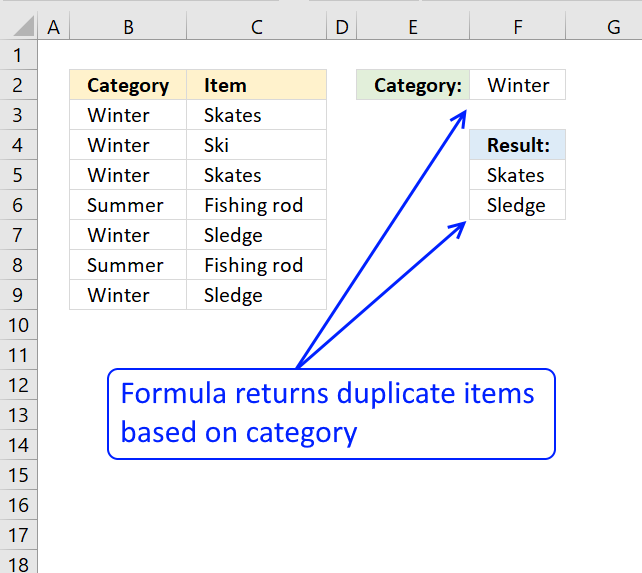The following image shows you a data set in columns B and C. The formula in cell E2 extracts a list of duplicates sorted from A to Z based on a condition in cell F2.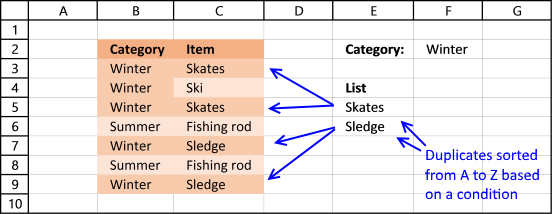"Fishing rod" is a duplicate value in column C but it is not in category "Winter", however "Skates" and "Sledge" are duplicates and in category "Winter.

## 1. Extract a list of alphabetically sorted duplicates based on a condition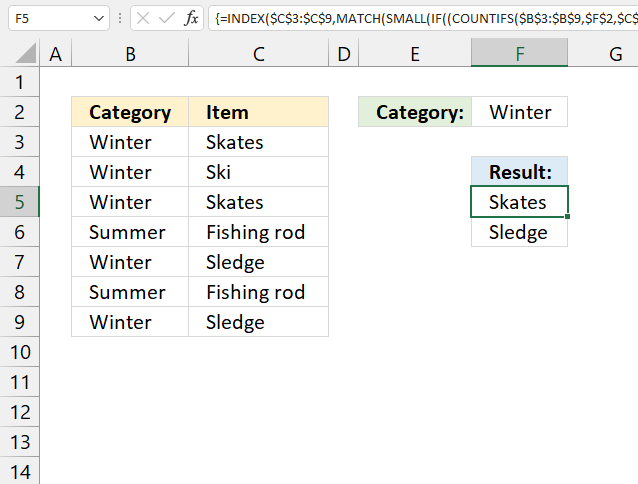Array formula in cell E5:

=INDEX(\$C\$3:\$C\$9, MATCH(SMALL(IF((COUNTIFS(\$B\$3:\$B\$9, \$F\$2, \$C\$3:\$C\$9, \$C\$3:\$C\$9)>1)*(COUNTIF(\$E\$4:E4, \$C\$3:\$C\$9)=0), COUNTIF(\$C\$3:\$C\$9, "<"&\$C\$3:\$C\$9), ""), ROWS(\$A\$1:A1)), COUNTIF(\$C\$3:\$C\$9, "<"&\$C\$3:\$C\$9), 0))

### 1.1 How to enter an array formula

1. Copy (Ctrl + c) above formula
2. Double press with left mouse button on cell E5
3. Paste formula to cell E5
4. Press and hold CTRL + SHIFT simultaneously
5. Press Enter once
6. Release all keys

The formula now looks like this: {=arrayformula}

Don't enter the curly brackets yourself, they appear automatically.

### 1.2 Explaining formula in cell E5

You can easily follow along, select cell E5. Go to tab "Formulas" on the ribbon and press with left mouse button on the "Evaluate Formula" button.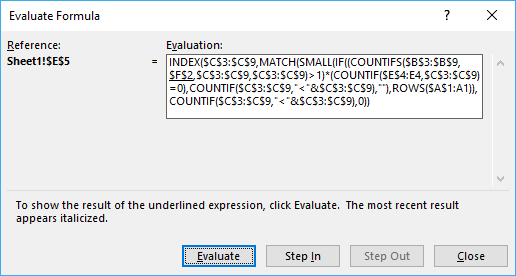Then press with left mouse button on "Evaluate" button to move to next step.

#### Step 1 - Check if category matches condition

COUNTIFS(\$B\$3:\$B\$9, \$F\$2, \$C\$3:\$C\$9, \$C\$3:\$C\$9)>1

becomes

COUNTIFS(\$B\$3:\$B\$9, \$F\$2, \$C\$3:\$C\$9, \$C\$3:\$C\$9)>1

becomes

COUNTIFS({"Winter"; "Winter"; "Winter"; "Summer"; "Winter"; "Summer"; "Winter"},"Winter",{"Skates"; "Ski"; "Skates"; "Fishing rod"; "Sledge"; "Fishing rod"; "Sledge"},{"Skates"; "Ski"; "Skates"; "Fishing rod"; "Sledge"; "Fishing rod"; "Sledge"})>1

and returns

{2;1;2;0;2;0;2}

#### Step 2 - Check if count is larger than 1

This allows us to filter duplicate values.

COUNTIFS(\$B\$3:\$B\$9, \$F\$2, \$C\$3:\$C\$9, \$C\$3:\$C\$9)>1

becomes

{2;1;2;0;2;0;2}>1

and returns {TRUE; FALSE; TRUE; FALSE; TRUE; FALSE; TRUE}

#### Step 3 - Count previous values in list

COUNTIF(\$E\$4:E4, \$C\$3:\$C\$9)

returns {0;0;0;0;0;0;0}

#### Step 4 - Check if they have not been shown before

This makes sure that previous values in column E are not repeated.

COUNTIF(\$E\$4:E4, \$C\$3:\$C\$9)>0

becomes

{0;0;0;0;0;0;0}=0

and returns {TRUE; TRUE; TRUE; TRUE; TRUE; TRUE; TRUE}

#### Step 5 - Multiply arrays

(COUNTIFS(\$B\$3:\$B\$9, \$F\$2, \$C\$3:\$C\$9, \$C\$3:\$C\$9)>1)*(COUNTIF(\$E\$4:E4, \$C\$3:\$C\$9)=0)

becomes

{TRUE; FALSE; TRUE; FALSE; TRUE; FALSE; TRUE}*{TRUE; TRUE; TRUE; TRUE; TRUE; TRUE; TRUE}

and returns {1;0;1;0;1;0;1}

#### Step 6 - Build an array of sort ranking numbers

COUNTIF(\$C\$3:\$C\$9, "<"&\$C\$3:\$C\$9) returns {2;4;2;0;5;0;5}

#### Step 7 - Return corresponding sort rank number

IF((COUNTIFS(\$B\$3:\$B\$9, \$F\$2, \$C\$3:\$C\$9, \$C\$3:\$C\$9)>1)*(COUNTIF(\$E\$4:E4, \$C\$3:\$C\$9)=0), COUNTIF(\$C\$3:\$C\$9, "<"&\$C\$3:\$C\$9), "")

becomes

IF({1;0;1;0;1;0;1},{2;4;2;0;5;0;5}, "")

and returns {2;"";2;"";5;"";5}

#### Step 8 - Find the n-th smallest number

SMALL(IF((COUNTIFS(\$B\$3:\$B\$9, \$F\$2, \$C\$3:\$C\$9, \$C\$3:\$C\$9)>1)*(COUNTIF(\$E\$4:E4, \$C\$3:\$C\$9)=0), COUNTIF(\$C\$3:\$C\$9, "<"&\$C\$3:\$C\$9), ""), ROWS(\$A\$1:A1))

becomes

SMALL({2;"";2;"";5;"";5}, ROWS(\$A\$1:A1))

becomes

SMALL({2;"";2;"";5;"";5}, 1)

and returns 2.

#### Step 9 - Get the positions of values in the array

MATCH(SMALL(IF((COUNTIFS(\$B\$3:\$B\$9, \$F\$2, \$C\$3:\$C\$9, \$C\$3:\$C\$9)>1)*(COUNTIF(\$E\$4:E4, \$C\$3:\$C\$9)=0), COUNTIF(\$C\$3:\$C\$9, "<"&\$C\$3:\$C\$9), ""), ROWS(\$A\$1:A1)), COUNTIF(\$C\$3:\$C\$9, "<"&\$C\$3:\$C\$9), 0)

becomes

MATCH(2, COUNTIF(\$C\$3:\$C\$9, "<"&\$C\$3:\$C\$9), 0)

becomes

MATCH(2, {2;4;2;0;5;0;5}, 0)

and returns 1.

#### Step 10 - Return value in data set based on coordinate

INDEX(\$C\$3:\$C\$9, MATCH(SMALL(IF((COUNTIFS(\$B\$3:\$B\$9, \$F\$2, \$C\$3:\$C\$9, \$C\$3:\$C\$9)>1)*(COUNTIF(\$E\$4:E4, \$C\$3:\$C\$9)=0), COUNTIF(\$C\$3:\$C\$9, "<"&\$C\$3:\$C\$9), ""), ROWS(\$A\$1:A1)), COUNTIF(\$C\$3:\$C\$9, "<"&\$C\$3:\$C\$9), 0))

becomes

INDEX(\$C\$3:\$C\$9, 1)

becomes

INDEX({"Skates";"Ski";"Skates";"Fishing rod";"Sledge";"Fishing rod";"Sledge"}, 5)

and returns "Skates" in cell E5.

## 2. Extract a list of alphabetically sorted duplicates based on a condition - Excel 365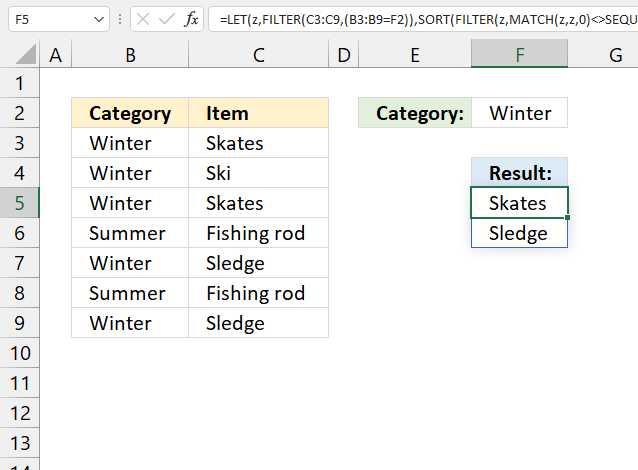Formula in cell F5:

=LET(z, FILTER(C3:C9, B3:B9=F2), SORT(FILTER(z, MATCH(z, z, 0)<>SEQUENCE(ROWS(z)))))

### 2.1 Explaining formula in cell F5

#### Step 1 - Check condition

The equal sign checks if the condition is equal to values in cell range B3:B9.

B3:B9=F2

becomes

{"Winter";"Winter";"Winter";"Summer";"Winter";"Summer";"Winter"}="Winter"

and returns

{TRUE; TRUE; TRUE; FALSE; TRUE; FALSE; TRUE}

#### Step 2 - Filter values based on criteria

The FILTER function filters values in a given cell range based on a condition or criteria.

FILTER(C3:C9, B3:B9=F2)

becomes

FILTER({"Skates"; "Ski"; "Skates"; "Fishing rod"; "Sledge"; "Fishing rod"; "Sledge"}, {TRUE; TRUE; TRUE; FALSE; TRUE; FALSE; TRUE})

and returns

{"Skates"; "Ski"; "Skates"; "Sledge"; "Sledge"}.

#### Step 3 - Assign a formula to a name

The LET function lets you assign a formula to a name. This reduces the formula size significantly.

FILTER(C3:C9, (B3:B9=F2) is assigned to z.

LET(z, FILTER(C3:C9, (B3:B9=F2)), formula)

#### Step 3 - Match filtered values

The MATCH function returns a number representing the relative position of an item in an array or cell range.

MATCH(z, z, 0)

becomes

MATCH({"Skates";"Ski";"Skates";"Sledge";"Sledge"}, {"Skates";"Ski";"Skates";"Sledge";"Sledge"}, 0)

and returns {1; 2; 1; 4; 4}.

#### Step 4 - Create a sequence based on the number of filtered values

The SEQUENCE function returns a sequence of numbers.

SEQUENCE(rows, [columns], [start], [step])

SEQUENCE(ROWS(z))

becomes

SEQUENCE(5)

and returns {1; 2; 3; 4; 5}.

#### Step 5 - Check for duplicates

The less than and greater than characters return TRUE if the numbers don't match indicating that the value is a duplicate.

MATCH(z, z, 0)<>SEQUENCE(ROWS(z))

becomes

{1; 2; 1; 4; 4}={1; 2; 3; 4; 5}

and returns {TRUE; TRUE; FALSE; TRUE; FALSE}.

#### Step 6 - Filter values

FILTER(z, MATCH(z, z, 0)<>SEQUENCE(ROWS(z)))

becomes

FILTER({"Skates"; "Ski"; "Skates"; "Sledge"; "Sledge"}, {TRUE; TRUE; FALSE; TRUE; FALSE})

and returns {"Skates";"Sledge"}.

#### Step 6 - Sort array

The SORT function sorts values in a cell range or array from A to Z.

SORT(FILTER(z, MATCH(z, z, 0)<>SEQUENCE(ROWS(z))))

becomes

SORT({"Skates";"Sledge"})

and returns {"Skates";"Sledge"}.